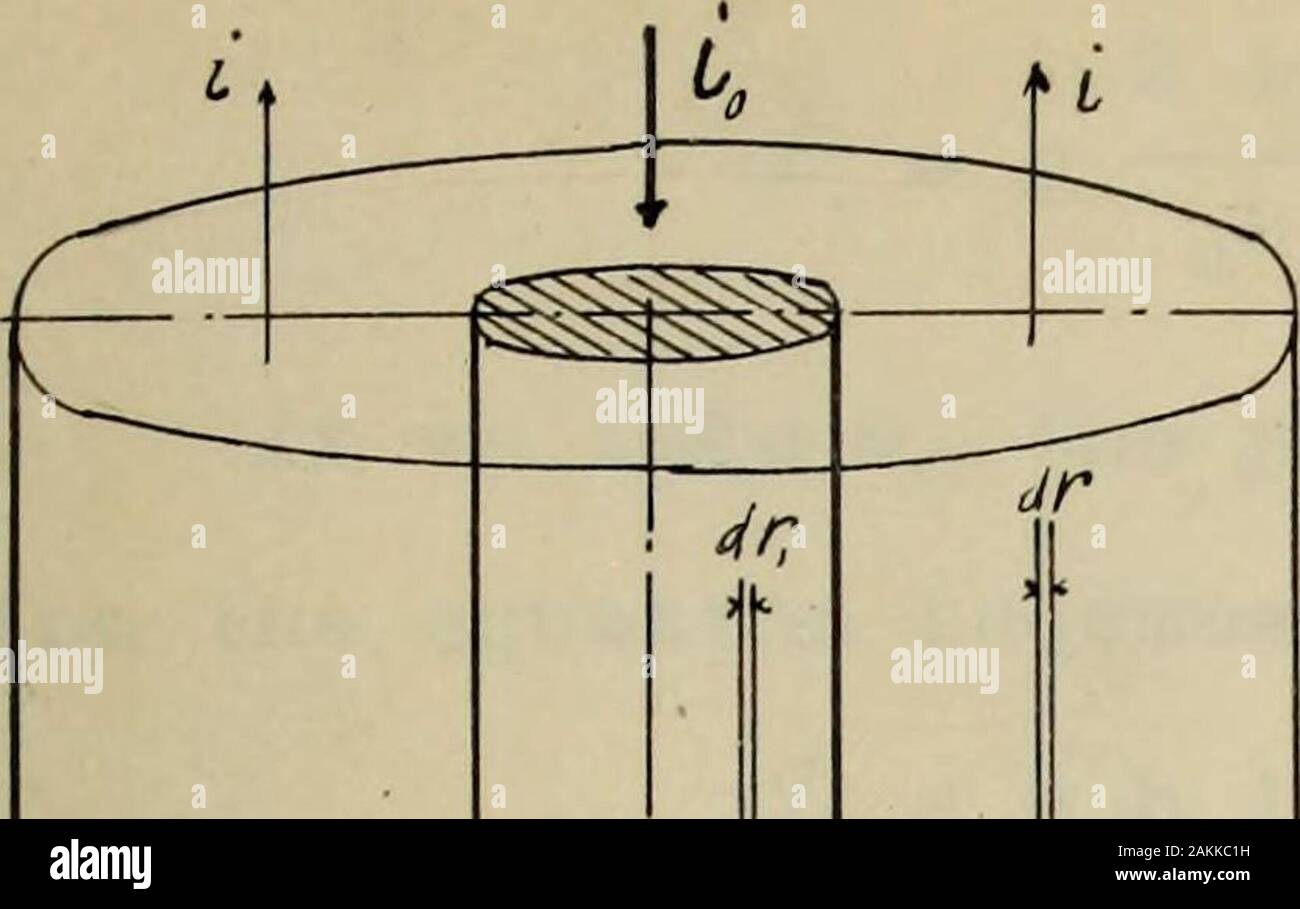# Lightning and lightning protections . Letus assume that this inverse displacement current is distributed 12 uniformly over the entire area. And let i^ = the whole current down axis. Here we have an equalinverse displacement current over the entire area (b2- a2), so thiithat the density of its distribution is j iQ = (b* - a2)j r = variable radius,dr = infinitesimal value of r. Taking a unit of magnetic force the circle whose radius r,the work done by the force is W = H2*r = 4n:(iQ i) The intensity of this furce at any distance out-side of theconductor 2 (i0 - i) H = r where i, the displacemen## Image details

Contributor:

The Reading Room / Alamy Stock Photo

Image ID:

2AKKC1H

File size:

Releases:

Model - no | Property - noDo I need a release?

Dimensions:

1992 x 1255 px | 33.7 x 21.3 cm | 13.3 x 8.4 inches | 150dpi

This image is a public domain image, which means either that copyright has expired in the image or the copyright holder has waived their copyright. Alamy charges you a fee for access to the high resolution copy of the image.

This image could have imperfections as it’s either historical or reportage.

Lightning and lightning protections . Letus assume that this inverse displacement current is distributed 12 uniformly over the entire area. And let i^ = the whole current down axis. Here we have an equalinverse displacement current over the entire area (b2- a2), so thiithat the density of its distribution is j iQ = (b* - a2)j r = variable radius, dr = infinitesimal value of r. Taking a unit of magnetic force the circle whose radius r, the work done by the force is W = H2*r = 4n:(iQ _ i) The intensity of this furce at any distance out-side of theconductor 2 (i0 - i) H = r where i, the displacement recovery current which lies nearerto the^than r. This is acculate for the distribution of the current doesnot matter as long as it is in coaxial cylinders. The portionsexternal to r have no effect. On the hypothesis of uniform distribution i = tl (r2- a2 ) j 2 2r - a b^- a^ 2 iQ(b2 - r2) r (b2 - a.2) .The total magnetic induction in the cylindrical space outside of the conductor or simply the magnetic flux at the externalconductor, . a ? &lt;pe = lh dr H = h/H * r*? 13 b ~ a a r h 2i b2dr b2~ a2 h 2i0 r , 2 2b - a b^logr - h 2i b2- — [b2io^ - — — ] - a^k a 2 2 J dr « /r a 2r^ r 2 b2 2 a 2 2 a is small compared with b that it isusual way negligible and we have: &lt;Pt = h i0[21og^ - 1 1 ... (IV)a ^ If weyu, the permiability of air in consideration the equa-tion (II) becomes , • . „ b (ivM. Cpt= u h i0[2 log lj Now we must calculate the flux inside of the conductor. Thecurrent density, j 1 7CBB Taking the radius inside of the conductor, thocurrent at 1 the region, call it ir which is i07t r2 r2= j*r2 = — = i a^ 2 ir H = = 2i 7L a2rl o rx a- . The magnetic flux inside of the cylinder, C#.= Jh drx H = hj2 g- drx V -1 14 2 i„ r 2i* h in a2 2 a2 =h i (V) If we take yUG the permiability of the conductor in consider*.ation the equation becomes = uQh i0 (V) Total magnetic flux, t b b (V = iQ h(2 log- - 1) h = inh 2 log - . (VI) a a with the permiabilities the equation bec

Save up to 70% with our image packs